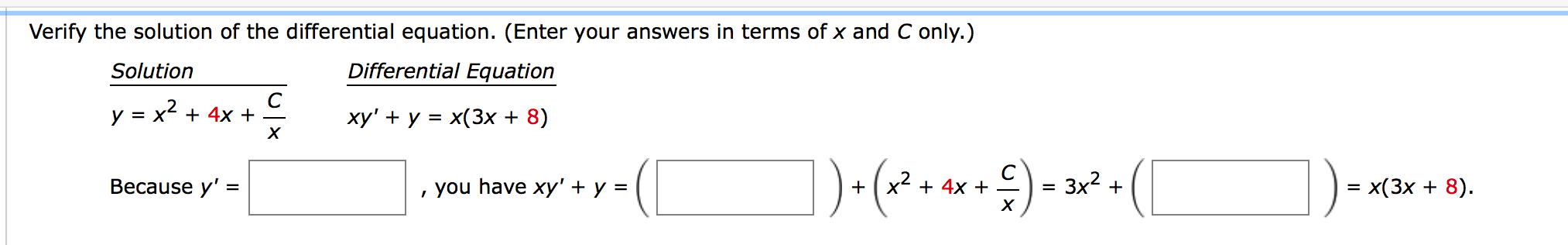# Verify the solution of the differential equation. (Enter your answers in terms of x and C only.) Solution Differential Equation y = x2 + 4x + xy' + y = x(3x + 8) Весause y' %3 , you have xy' + y = x + 4x + 3x2 + 3D x(3х + 8). + %D

Questionhelp_outlineImage TranscriptioncloseVerify the solution of the differential equation. (Enter your answers in terms of x and C only.) Solution Differential Equation y = x2 + 4x + xy' + y = x(3x + 8) Весause y' %3 , you have xy' + y = x + 4x + 3x2 + 3D x(3х + 8). + %D fullscreen

### Want to see this answer and more?

Experts are waiting 24/7 to provide step-by-step solutions in as fast as 30 minutes!*

*Response times may vary by subject and question complexity. Median response time is 34 minutes for paid subscribers and may be longer for promotional offers.
Tagged in
Math
Calculus

### Differential Equations